# Influence of Buoyant Forces on Magnetohydrodynamics (MHD) Blood Flow with an Interaction of Thermal Radiation

Influence of Buoyant Forces on Magnetohydrodynamics (MHD) Blood Flow with an Interaction of Thermal Radiation
Department of Mathematics, Siksha O Anusandhan University, Bhubaneswar 751030, India

Corresponding Author Email:
Page:
71-80
|
DOI:
https://doi.org/10.18280/mmep.080109
25 October 2019
|
Accepted:
2 December 2020
|
Published:
28 February 2021
| Citation

OPEN ACCESS

Abstract:

In this study, the heat and mass transfer of the blood flow, particularly in a capillary tube having a porous lumen and permeable wall in the presence of external magnetic field are considered. The velocity, temperature and concentration of blood flow become unsteady due to the time dependence of the stretching velocity, surface temperature and surface concentration. The thermal and mass buoyancy effect on blood flow, heat transfer and mass transfer are taken into account in the presence of thermal radiation. This analysis is very much useful in the treatment of cardiovascular disorders. The equations governing the flow under some assumptions are complex in nature, but capable of presenting the realistic model of blood flow using the theory of boundary layer approximation and similarity transformation. First, the system of coupled partial differential equations (PDEs) is converted into a system of coupled ordinary differential equations (ODEs). Then the solutions are obtained by Runge-Kutta method of 4thorder with shooting technique. The effects of various parameters such as Hartman number, radiation parameter, unsteadiness parameter, permeable parameter, thermal buoyancy parameter, Prandtl number, mass buoyancy parameter, velocity slip parameter, thermal slip parameter, Schmidt number on velocity, temperature, concentration, skin friction, Nusselt number and Sherwood number are depicted through graphs. Local Sherwood number enhances because of increase in Schmidt number. Moreover, some of the important results, which are discussed in the present study and have an impact on diseases like hyperthermia, stoke and moyamoya in human body.

Keywords:

thermal radiation, slip flow, permeability, thermal and mass buoyancy, Runge-Kutta method

1. Introduction

The blood flow with heat and mass transfer plays a vital role in the field of biomedical engineering and biomedical science. Blood is a fluid that transports oxygen and other essential substances throughout the human body. Blood which is a very important liquid in human limbs and that execute numerous operations like removal of carbon dioxide, transport of nutrients, removal of metabolic product, oxygen transport. Circulation of blood is classified as circulation of capillary, circulation of systemic and microcirculation. The flow of blood can be quantified by various processes such as video capillary, microscopic and laser doppler anemometry. Moreover, it plays a vital role in digestion and endocrine system functions in the body. Blood absorbs and distributes heat throughout the body to maintain homeostasis. The influence of heat and mass transfer has received a lot of attention due to various applications in human thermotherapy, cardiovascular disease, conduction process in tissues, radiation between the surface and its environment. Heat transfer in individual blood vessels for three different configurations investigated by Chato . Misra and Shit  discussed the role of velocity slip in blood flow through stenosed arteries. Barozzi and Dumas  presented a study of convective heat transfer in blood vessels at the wall of the vessel numerically. They found that a more increase in the rate of heat transfer in the small vessels due to comparatively low rheological behavior of blood. Convective heat transfer of fully developed two- fluid model at the time of blood flow in small tubes with constant heat flux studied by Wang . He found that the less viscous cell-free wall layers the increases the flow and promotes the heat transfer and large blood vessels can produce steep temperature gradients in heated tissues during hypothermia. Nakayama and Kuwahara  presented a volume averaging theory (VAT) model to derive bioheat transfer equations for blood flows and its surrounding biological tissue in a saturated porous medium. A two-phase model consists of a central core of suspended erythrocytes and a cell-free layer surrounding the core for the blood flow in a narrow tube described by Sharan and Popel . Some experimental investigations on the role of blood in the presence of heat source/sink in human organs carried by Ducharme and Tikuisis . The experimental study of temperature variation along artery-vein pairs in rat hind limb investigated by He et al. .

The mass transfer plays a vital role in the diffusion process such as mass diffusion in tissues and drug synthesis in the human body. Srinivas et al.  studied theoretically the unsteady MHD flow of heat and mass transfer in porous blood vessel over a permeable stretching sheet with slip conditions. Akbar  discussed the effect of heat and mass transfer of Prandtl fluid model in a tapered stenosis artery and found that the velocity decreases due to an increase in Prandtl fluid parameters and the maximum height of the stenosis. Ellahi et al.  considered the effects of heat and mass transfer on peristaltic flow of viscous fluid in a non-uniform rectangular duct. Moreover, they have studied the peristaltic motion of blood for Ree-Eyring fluid pattern under the action of exterior magnetic field influence on the heat and mass transfer.

Recently, Amiri et al.  have presented a 3-D numerical simulation of non-Newtonian blood flow through femoral artery bifurcation with a moderate arteriosclerosis: investigating Newtonian/non-Newtonian flow and its effects on elastic vessel walls. In the present study they have considered, a fluid structure interaction simulation of the blood flow in the femoral artery with a small occlusion. They have also accounted for unstable blood flow at the inlet specifically in leg blood vessels. The important observations in respects of hemodynamic parameters such as velocity profiles, vortices shape and pressure drops are noteworthy. Their study reveals that employing Newtonian models for blood flow does not head the promising result, particularly through occluded passage and beyond, in comparison with non –Newtonian model. The result is more relevant to blood vessels modelled as an isotropic elastic wall.

Very recently Zigta  has considered the effect of thermal radiation and chemical reaction MHD flow of blood in stretching permeable vessel. This topic is closer to the present analysis, irrespective of solute concentration, thermal radiation and time dependent magnetic field intensity but the study does not account for the two additional free convective body forces due to mass and thermal buoyancy affecting the flow. That become the new findings of the present analysis. Zigta  has observed that the temperature of the blood increases at the vessel wall as the Prandtl number and Hartman number increase. Concentration of blood decreases as Schmidt number increases.

On the basis of all the discussion Misra and Sinha  examined the MHD blood flow in a tube and its artery is porous and the surface is permeable. Since the velocity in stretching motion and temperature of surface are function of time so that occur the unsteadiness in the flow field and temperature field, velocity slip, thermal radiation, thermal slip condition and mass slip conditions are taken into consideration. we have interested to study the unsteady behaviour of MHD fluid flow, heat and mass transfer of blood in certain thin permeable capillary vessel which has significant use in many clinical sciences, mainly in thermal therapy. In this study, we also proposed to investigate the impact of thermal buoyancy and mass buoyancy on MHD flow of blood in a permeable capillary with stretching motion in the presence of radiation. The governing equations of the flow mechanism are streamlined under the consideration of lengthy wave length. The impact of magnetic field is also taken into consideration by the use of Ohms law. The transformed coupled PDEs are work out numerically. The influence of the embedding parameters of concern problem is interpreted using the graphs.

2. Analysis

Unsteady flow of blood with radiation through a narrow tube with permeable surface under the action of an unsteady magnetic field B(t) which applied in a transverse direction is considered. The effect of thermal buoyancy, mass buoyancy during the flow will also considered in the analysis. Let us consider the flow is along the x-axis and y-axis is perpendicular to it. It is considered that the sheet is moving with an initial velocity $U_{w}(x, t)$ along the direction of x-axis, where the sheet and ambient temperatures are assumed as $T_{w}(x, t), T_{\infty}$ respectively. However, the concentration near the sheet and far away from it are treated as $C_{w}(x, t), C_{\infty}$. The geometry of flow model is shown in Figure 1. Since the flow of blood is MHD in nature, so that magnetic Reynolds number is very small. Therefore, the induced magnetic field is dominated by the applied magnetic field which is not considered in our present analysis. Although the flow in the system is 3-D in nature, but flow in micro vessels can be considered to be 2-dimensional in many problems. Here the flow of blood is considered as passing through a narrow porous medium with permeable surface. So, the mathematical formulation that characterises the flow of blood, heat and mass transfer phenomena under different boundary conditions for this study can be represented in the following form.

## 1.pngFigure 1. Geometrical interpretation of the physical problem

$\frac{\partial u}{\partial x}+\frac{\partial v}{\partial y}=0$     (1)

$\frac{\partial u}{\partial t}+u \frac{\partial u}{\partial x}+v \frac{\partial u}{\partial y}=v \frac{\partial^{2} u}{\partial y^{2}}-\frac{v}{k_{1}(t)} u-\frac{\sigma B^{2}(t)}{\rho} u$

$+g \beta\left(T-T_{\infty}\right)+g \beta^{*}\left(C-C_{\infty}\right)$     (2)

$\frac{\partial T}{\partial t}+u \frac{\partial T}{\partial x}+v \frac{\partial T}{\partial y}=\frac{k}{\rho c_{p}} \frac{\partial^{2} u}{\partial y^{2}}-\frac{1}{\rho c_{p}} \frac{\partial q_{r}}{\partial y}$    (3)

$\frac{\partial C}{\partial t}+u \frac{\partial C}{\partial x}+v \frac{\partial C}{\partial y}=D \frac{\partial^{2} C}{\partial y^{2}}$     (4)

The wall being permeable, velocity, thermal and mass slip is taken into consideration and the boundary conditions are

$u=U_{w}+N \mu \frac{\partial u}{\partial y}=U_{w}+u_{s}, T=T_{w}+K \frac{\partial T}{\partial y}=T_{w}+$$T_{s}, v=V_{w} C=C_{w}+C_{s}=C_{w}+K_{1} \frac{\partial C}{\partial y}, at y=0 (5) u \rightarrow 0, C \rightarrow \infty, T \rightarrow \infty, at y \rightarrow \infty Wall of the capillary which is expressed by V_{w}=-\sqrt{\frac{U_{w} v}{x}} f(0) (6) From the above equation, the flow at the surface of the tube with a velocity Vw, where Vw is positive for injection and Vw is negative for suction. Unsteady permeability parameter of blood in the capillary tube, which is a function of time being defined in Eq. (2) is k_{1}(t)=k_{2}(1-c t) . {\text { The }} velocity, mass, thermal slip factor in equation (5) are N=N_{0} \sqrt{1-c t}, K_{1}=K_{1_{0}} \sqrt{1-c t}, K=$$K_{0} \sqrt{1-c t}$ respectively. The absence of slip conditions can be evaluated by substituting $N=K_{1}=K=0$.

In order to get similarity solution, we consider the surface temperature Tw(x,t), the velocity of blood Uw(x,t), the surface mass Cw(x,t), magnetic strength at t=0 within a capillary $B(t)=\frac{B_{0}}{1-c t}$ magnetic strength at t=0 within a capillary are in the following form

$T_{w}(x, t)=T_{\infty}+\frac{b x}{1-c t}, U_{w}(x, t)=\frac{a x}{1-c t}$ $C_{w}(x, t)=\mathrm{C}+\frac{d x}{1-c t}$ and $B(t)=\frac{B_{0}}{1-c t}$     (7)

Here $b, a, c, d$ and $B_{0}$ are constants, such that $b \geq 0, a>$ $0, d \geq 0$ and $c t<1$.

With the help of Rosseland approximation, the relative heat flux is represented by

$q_{r}=-\frac{\partial T^{4}}{\partial y} \frac{4 \sigma^{*}}{3 k^{*}}$      (8)

Now let us assume that the temperature difference along the blood vessel is very negligible and by the help of expansion of Taylor series and truncating the higher order terms we have

$T^{4}=T_{\infty}^{3}\left(4 T-3 T_{\infty}\right)$     (9)

Here $T_{\infty}$ stands for ambient temperature.

The following similarity transformations with suitable choice of stream function $\psi$ are used in Eqns. (1), (2), (3) and (4),

\begin{aligned} \theta(\eta)=\frac{T-T_{\infty}}{T_{w}-T_{\infty}}, f(\eta) &=\frac{\psi}{\sqrt{v x U_{W}}}, \phi(\eta)=\frac{C-C_{\infty}}{C_{w}-C_{\infty}} \\ \eta &=\sqrt{\frac{U_{w}}{x v}} y \end{aligned}     (10)

where, $u=\frac{\partial \psi}{\partial y}$ and $v=-\frac{\partial \psi}{\partial x}$.

We obtained a system of coupled nonlinear ordinary differential equation

$f^{\prime \prime \prime}+f^{\prime \prime}(f-A(\eta / 2))-f^{\prime}\left(M^{2}+A+1 / k_{3}\right)-$

$f^{\prime 2}+\lambda_{1} \theta+\lambda_{2} \phi=0$    (11)

$(N r+1) \theta^{\prime \prime}+\operatorname{Pr} f \theta^{\prime}-\operatorname{Pr} f^{\prime} \theta$

$-\operatorname{APr}\left(\frac{1}{2} \eta \phi^{\prime}+\phi\right)=0$      (12)

$\phi^{\prime \prime}+\operatorname{Sc} \phi^{\prime}(f-(A / 2) \eta)-\operatorname{Sc} \phi\left(f^{\prime}+A\right)=0$    (13)

where the unknown physical quantities like $A, \operatorname{Pr}, S c, M, k_{3}, N r, \lambda_{1}$ and $\lambda_{2}$ are defined in nomenclature with their values given as follows:

$\operatorname{Pr}=\frac{\mu c_{p}}{k}, S c=\frac{D}{v}, \lambda_{1}=\frac{g \beta b(1-c t)}{a^{2}}, \lambda_{2}=\frac{g \beta^{*} d(1-c t)}{a^{2}}$

$A=\frac{c}{a}, M=B_{0} \sqrt{\frac{\sigma}{\rho a}}, k_{3}=\frac{a k_{2}}{v}, N r=\frac{16 \sigma^{*} T_{\infty}^{3}}{3 k^{*} k}$

With the help of (5), we have the transformed boundary conditions for solving equations from (11) to (13) are

$f(0)=S, f^{\prime}(0)=S_{f} f^{\prime \prime}(0)+1, \theta(0)=S_{t} \theta^{\prime}(0)+$1 and $\phi(0)=S_{m} \phi^{\prime}(0)+1$

$f^{\prime}(\eta) \rightarrow 0, \theta(\eta) \rightarrow 0, \phi(\eta) \rightarrow 0$ as $\eta \rightarrow \infty$     (14)

where, $S_{t}, S_{m}$ and $S_{f}$ stands for dimensionless thermal, mass and velocity slip factor respectively. In the above mathematical expression, the primes stand for the rate of change of dimensionless function with a corresponding change of physical variable $\eta .$ Putting $A=0$ in Eqns. (11), (12) and (13) the system of equation for unsteady problem has changed to that of steady one.

$S_{t}=K_{0} \sqrt{\frac{b}{v}}, S_{m}=K_{1_{0}} \sqrt{\frac{a}{v}}, S_{f}=N_{0} \rho \sqrt{a v}$     (15)

3. Skin-Friction Coefficient, Nusselt Number and Sherwood Number

The behaviour of physical parameter in the fluid flow like wall shear stress, heat and mass transfer rate of interest are defined by

$C_{f}=\frac{2 \tau_{w}}{\rho U_{w}^{2}}$    (16)

where the surface wall skin friction is defined as

$\tau_{w}=\mu\left(\frac{\partial u}{\partial y}\right)_{y=0}$    (17)

and the mass flux (mw) and the heat flux (qw) at the surface wall are defined as

$q_{w}=-K\left(\frac{\partial T}{\partial y}\right)_{y=0}$ and $m_{w}=-D\left(\frac{\partial c}{\partial y}\right)_{y=0}$     (18)

Now Nux is the local Nusselt number and Shx is the local Sherwood number, which are expressed as

$N u_{x}=\frac{x q_{w}}{T_{w}-T_{\infty}}=-R e_{x}^{\frac{1}{2}} \theta^{\prime}(0)$ and

$S h_{x}=\frac{x m_{w}}{D\left(C_{w}-C_{\infty}\right)}=-R e_{x}^{\frac{1}{2}} \phi^{\prime}(0)$     (19)

where, $\mathrm{Re}_{x}^{\frac{1}{2}}=K \sqrt{\frac{x U_{W}}{v}}$ local Reynolds number.

4. Computational Method

The non-linear coupled ordinary differential Eqns. (11)-(13) along with prescribed boundary conditions (14) form a two-point BVPs. First, these equations are converted into an IVPs and then solved numerically by Runge Kutta method of order 4 with shooting technique. Let us consider

$f^{\prime}=p, q=p^{\prime}, q^{\prime}=-\left(q\left(p-A \frac{\eta}{2}\right)\right)-p\left(M^{2}+A+\right.$

$\left.\frac{1}{k_{2}}\right)-p^{2}+\lambda_{1} r+\lambda_{2} s$     (20)

$\theta^{\prime}=r, r^{\prime}$$\left.=-(\operatorname{Pr}(1+N r))\left(f r-p \theta-A\left(\frac{\eta s^{\prime}}{2}+s\right)\right)\right) (21) \phi^{\prime}=s, s^{\prime}=-(S c)\left(f-(p+A) \phi-\frac{A}{2} s\right) (22) with the corresponding initial conditions are f(0)=S, p(0)=S_{f} q(0)+1, \theta(0)=S_{t} r(0)+1 \quad and \phi(0)=S_{m} s(0)+1 (23) The initial conditions (23) are not sufficient, soq(0), r(0) and s(0) values are required to solve the Eqns. (20),(21) and (22) . These conditions are absent in the present problem. First, the initial values of q(0), r(0) and s(0) are chosen and using 4^{\text {th }} order Runge-Kutta method computed the values of f^{\prime}(\eta), \theta(\eta) and \phi(\eta) at a suitably large value of \eta. Again, the initial approximations are improved using Newton method to get an improved solution. Finally, the computed values of will converge to the boundary conditions f^{\prime}(\eta)= 0, \theta(\eta)=0 and \phi(\eta)=0. as \eta \rightarrow \infty. The computation has been carried out with step size \delta \eta=0.008. We have computed the values again the smaller value of \delta \eta but that does not give any moderate change in the computed results. This indicates the accuracy, stability and convergence of our numerical technique. 5. Results and Discussion Eqns. (11) (12) and (13) with the prescribed boundary conditions (14) have been solved numerically by 4th order Runge-Kutta method association with Shooting technique. From the experimental point of view, we are able to know that the blood of human being has been influenced by the physical parameters like viscosity (\mu), specific heat at constant pressure (cp), thermal conductivity (k) which are dependent upon time. Researcher Chato  has presented the following set of data for blood of human being at a temperature T=310^{\circ} \mathrm{K}, c_{p}=14.65 \mathrm{j} / \mathrm{kg}^{0} \mathrm{~K}, \mu=3200 \mathrm{~kg} / \mathrm{ms} \text { and } k=$$0.0022 \mathrm{j} / \mathrm{ms}^{0} \mathrm{~K}$. On the basis of all these above information's, we have evaluated that Pr=21 for blood of human being. The computations have been carried out for value of \begin{aligned} S &=0.5, k_{3}=0.4, A=1, S_{f}=2.5, S_{t}=1, S_{m}=1, \operatorname{Pr}=\\ 21, t &=0.1, N r=0.2, S c=1, \lambda_{1}=1, \lambda_{2}=1 \text { for Figure } 2-19 \end{aligned} for . The influence of magnetic parameter in absence of thermal and mass buoyancy on velocity distribution is depicted in Figure 2. It is observed that the velocity profile retards with rise in magnetic parameter. This is because of Lorentz force which acts in the opposite direction of flow of blood. The increase in generated Lorentz force reduces the blood velocity in the capillary tube. The figure also indicates that the velocity of blood flow in the capillary tube is reduced with an increase in the height from the lower wall. Results presented in Figure 2 which establishes the validity of the results obtained by comparing with Misra and Sinha .

## 2.png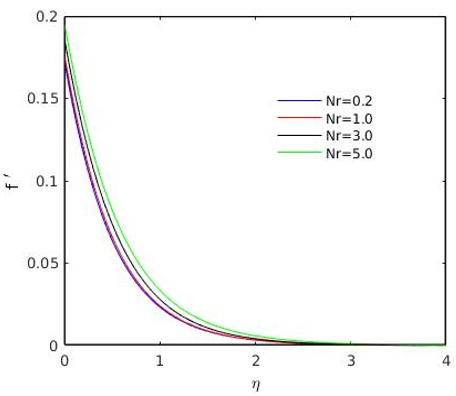Figure 2. Effect of M2 on velocity

## 3.png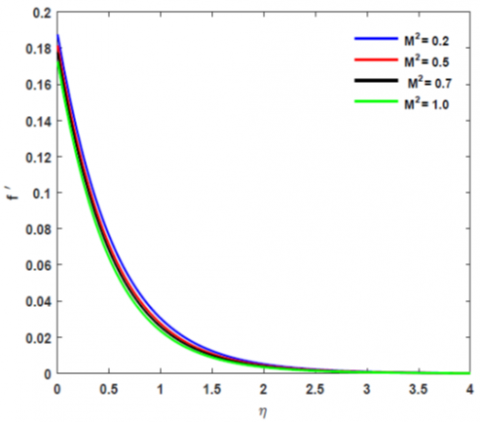Figure 3. Effect of Nr on velocity

## 4.pngFigure 4. Effect of A on velocity

## 5.png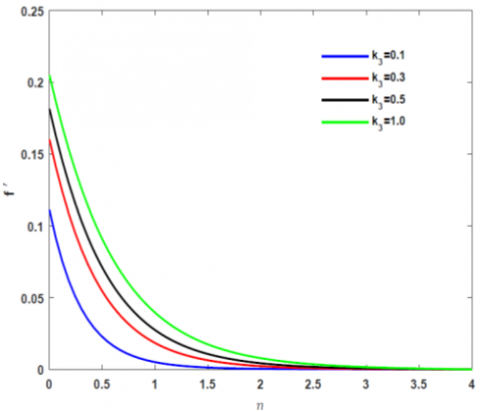Figure 5. Effect of k3 on velocity

Figure 3 depicts the influence of radiation parameter (Nr) over non-dimensional axial velocity. The effect of radiation by thermal process increases then the velocity of blood rises in the capillaries. In some pathological process, we can reduce the pain in the affected area by applying heat due to which blood clot breaks and starts to flow in a regular manner. The change of non-dimensional axial velocity for various values of unsteadiness parameter (A) has been depicted in Figure 4. The present figure shows that velocity of blood in the capillary falls down with rise up of the unsteadiness parameter (A) but one interesting property is marked here that the velocity of blood is very low in absence of (A). Increase in permeability parameter that tends to rise in axial velocity which is illustrated in Figure 5. In addition, small change in value of permeability parameter (k3) produces faster declination in axial velocity. Figure 6 represents the velocity profile for different values of buoyancy parameter (λ1). Now the velocity of blood rises toward the axis of the capillary line, when the value of thermal buoyancy parameter (λ1) go high within the region where flow assist (λ1>0), while it’s value drop-down when the thermal buoyancy parameter (λ1) rises within the region where the flow opposes (λ1<0). In physical science, the flow opposing region is meant for increase temperature of fluid or decrease temperature of stretching tube, while flow assisting region meant for decrease temperature of fluid or increase temperature of tube. The current picture further reveals that when the temperature of the surface wall of capillary tube decreases, the boundary layer thickness of velocity rises whereas when temperature of blood decreases, then the boundary layer thickness of physical quantity velocity increases. It has been noted that for the case when the temperature of surface wall of the capillary vessel increases with increase of λ1 then the velocity of blood becomes zero at inflection point. The blood flow velocity which flows towards the radial direction for different value of mass buoyancy parameter has been depicted in Figure 7. Buoyancy is an opposing force act in upward direction exerted by a fluid that opposes the weight of a mass object in the blood so the velocity of blood goes high, when the thermal buoyancy parameters rise up. The influence of the velocity slip factor and thermal slip factor on axial velocity has been described in Figure 8 and Figure 9 respectively. Figure 8 shows that the velocity in axial direction decreases when the velocity slip factor (Sf) increases. A similar effect is also observed for the mass slip factor (St) that has shown in Figure 9. It is noticed that for higher value of (Sf) and (St) the frictional resistance within the blood and surface wall is completely removed. Figure 10 to Figure 15 depict the temperature distribution for various values of thermal buoyancy parameter (λ1), mass buoyancy parameter (λ2), Hartmann number (M), Prandtl number (Pr) and radiation parameter (Nr). The influence of thermal buoyancy parameter and mass buoyancy parameter presented in Figure 10 and Figure 11 respectively. So, the flow velocity increases with an increase in thermal buoyancy parameter because of the contribution of gravity helps blood particles in attaining higher kinetic energy. Figure 12 represents the influence of magnetic parameter (M2) over distribution of temperature. This is an interesting fact to note that the temperature of the boundary layer of the capillary vessel increases due to the existence of a magnetic field. It is an important fact to see that an opposing type of force is well known as Lorentz force has been exhibited from an electrically conducting fluid subject to the action of magnetic field, which is normal to the surface. The forces have the capability to increase the temperature of blood flow.

## 6.pngFigure 6. Effect of λ1 on velocity

## 7.pngFigure 7. Effect of λ2 on velocity

## 8.png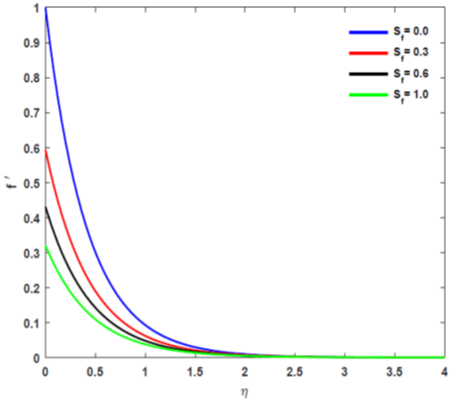Figure 8. Effect of Sf on velocity

## 9.pngFigure 9. Effect of St on velocity

## 10.png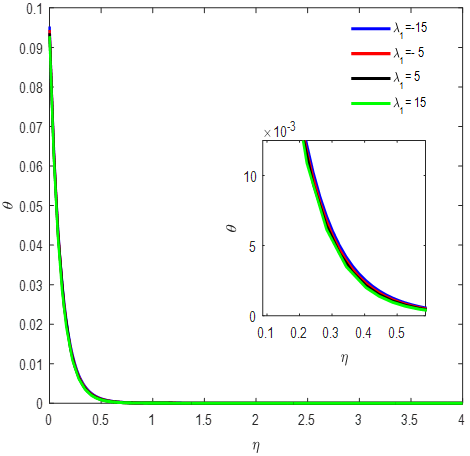Figure 10. Effect of λ1 on temperature

## 11.pngFigure 11. Effect of λ2 on temperature

## 12.pngFigure 12. Effect of M2 on temperature

## 13.pngFigure 13. Effect of Nr on temperature

The influence of thermal radiation over the temperature distribution has been depicted in Figure 13. The increase of thermal radiation at the time of blood flow in the capillary tube gives rise to the corresponding increase in the thickness of the boundary layer which has been highlighted in the figure. The variation of the temperature in the boundary layer due to the corresponding variation in the Prandtl number (Pr) has been shown in Figure 14. The present reveals that the Prandtl number rises up when the temperature of the boundary layer falls down. It is an important fact to notice that the thickness of the thermal boundary layer declines due to the increase of Prandtl number (Pr). It further marks that the temperature distribution at the surface wall increases due to increase in Prandtl number because when Prandtl number of blood is very high, then the thermal conductivity (k) let down and due to which heat conduction capacity decreases by reducing the thickness of boundary layer, therefore the rate of heat transfer at the surface of vessel increases. Figure 15 depicts the variation of temperature profile in blood for the various values of thermal slip factor (St). The temperature at any point in the flow decreases due to increase in the thermal slip parameter.

## 14.pngFigure 14. Effect of Pr on temperature

## 15.png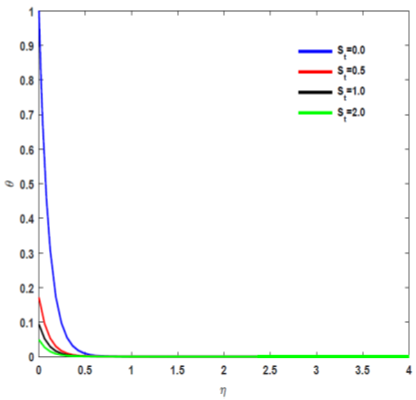Figure 15. Effect of St on temperature

## 16.png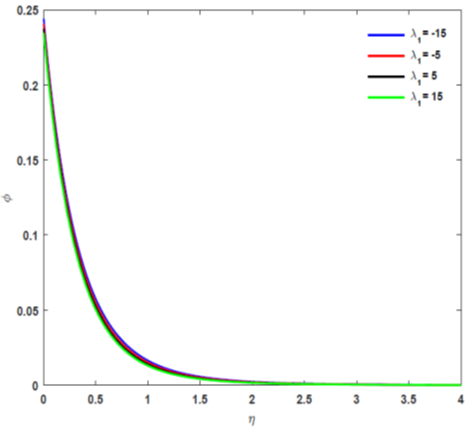Figure 16. Effect of λ1 on concentration

## 17.png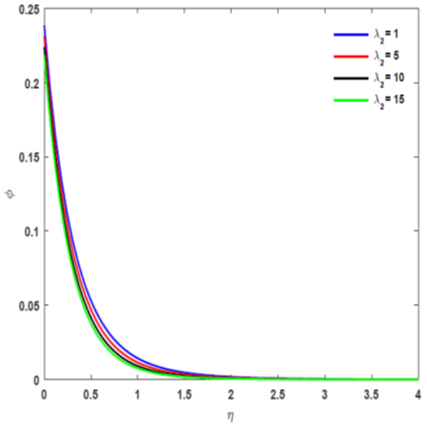Figure 17. Effect of λ2 on concentration

## 18.png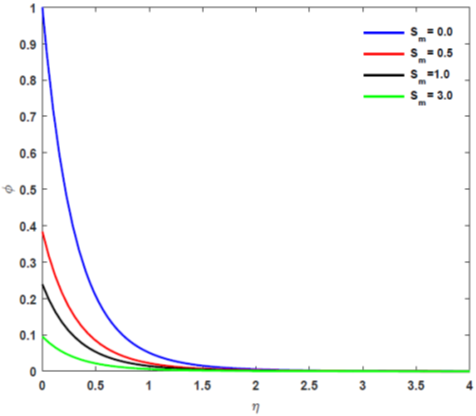Figure 18. Effect of Sm on concentration

## 19.pngFigure 19. Effect of Sc on concentration

## 20.png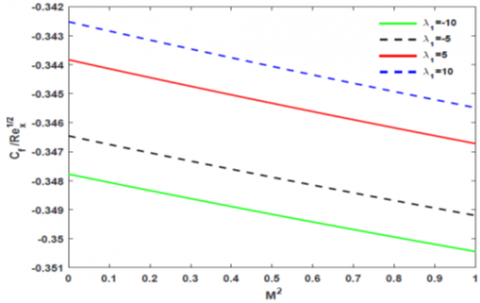## 22.png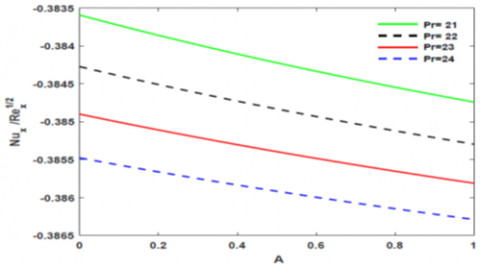Figure 22. Effect of Pr on Nusselt number with $A$ for $S=0.5, k_{3}=0.4, S_{f}=2.5, S_{t}=2.5, S_{m}=1, t=0.1, M=$$0.5, N r=0.2, S c=1, \lambda_{1}=1, \lambda_{2}=1 ## 23.png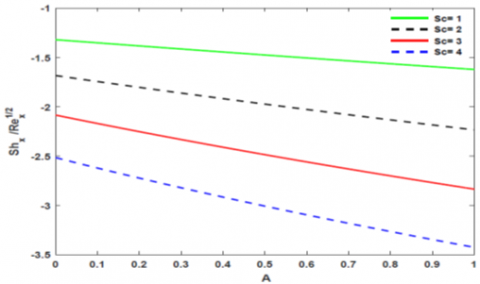Figure 23. Effect of S con Sherwood number with A for S=0.5, k_{3}=0.4, S_{f}=2.5, S_{t}=2.5, S_{m}=1, t=0.1$$M=0.5, N r=0.2, \operatorname{Pr}=21, \lambda_{1}=1, \lambda_{2}=1$

6. Conclusion

Unsteady blood flow with radiation through a narrow tube with permeable surface has been discussed in the presence of applied unsteady magnetic field. The influence of thermal and mass buoyancies was investigated in this model. The velocity of blood flow, temperature and mass concentration distributions along with dimensionless skin friction, Nusselt number and Sherwood number at the capillary for various values of parameters are studied and shown graphically. The model would be very much useful to medical engineering like human thermotherapy, cardiovascular diseases. We have established the accuracy of numerical results computed by Misra and Sinha  in our study. The important findings of the present study have outlined as given below:

By controlling the exterior magnetic field intensity, the velocity of the blood can be regulated and the velocity profile retards with rise in magnetic parameter. Moreover, when the velocity slip and thermal slip factor increases, then the velocity of blood towards the axial direction decreases.

It is the ability of thermal radiation to produce a meaningful variation in the temperature distribution of boundary layer. The thermal boundary layer thickness goes high to a great extent when an increase in thermal radiation and also the blood temperature along the boundary layer decreases if the Prandtl number of thermal slip factor increases.

The blood velocity towards the axis of tube goes high due to increase in permeability parameter. It is concluded that the amount of mass concentration inside the capillary tube falls down due to the increase of Schmidt number, thermal buoyancy parameter and mass buoyancy parameter.

The concentration distribution of blood at any position of the capillary tube decreases if the mass slip factor increases.

In future, the present paper will be helpful in determining experimental as well as theoretical analysis of more complicated blood flow model.

Nomenclature
 Ts thermal slip H heat transfer coefficient v velocity of blood in tube along the direction of normal us velocity- slip at the permeable wall u blood velocity along the axis of the tube B(t) unsteady magnetic field k2 permeability K thermal slip factor qr radiative heat flux N velocity slip factor M Hartman number A unsteadiness parameter Pr Prandtl number v kinematic coefficient of viscosity Sf velocity slip factor Nux local Nusselt number Sm mass slip factor $\eta$ distance Uw stretching velocity $\sigma$ electrical conductivity k* mean absorption coefficient Tw surface temperature cp specific heat at constant pressure Nr radiation parameter D mass diffusivity k3 permeability parameter k1(t) unsteady permeability parameter T temperature of blood at any point in the tube K1 mass-slip factor k thermal conductivity St thermal-slip factor vw injection or suction velocity $\sigma^{*}$ Stefan-Boltzmann constant Cf skin-friction coefficient Sh Sherwood number r radius of the artery line S injection or suction parameter $\rho$ density
References

 Chato, J.C. (1980). Heat transfer to blood vessels. Journal of Biomechanical Engineering, 102(2): 110-118. https://doi.org/10.1115/1.3138205

 Misra, J.C., Shit, G.C. (2007). Role of slip velocity in blood flow through stenosed arteries: A non-Newtonian model. Journal of Mechanics in Medicine and Biology, 7(3): 337-353. https://doi.org/10.1142/S0219519407002303

 Barozzi, G.S., Dumas, A. (1991). Convective heat transfer coefficients in the circulation. Journal of Biomechanical Engineering, 113(3): 308-313. https://doi.org/10.1115/1.2894889

 Wang, C.Y. (2008). Heat transfer to blood flow in a small tube. Journal of Biomechanical Engineering, 130(2): 024501-024501.

 Nakayama, A., Kuwahara, F. (2008). A general bioheat transfer model based on the theory of porous media. International Journal of Heat and Mass Transfer, 51(11-12): 3190-3199. https://doi.org/10.1016/j.ijheatmasstransfer.2007.05.030

 Sharan, M., Popel, A.S. (2001). A two-phase model for flow of blood in narrow tubes with increased effective viscosity near the wall. Biorheology, 38(5-6): 415-428.

 Ducharme, M.B., Tikuisis, P. (1994). Role of blood as heat source or sink in human limbs during local cooling and heating. Journal of Applied Physiology, 76(5): 2084-2094. https://doi.org/10.1152/jappl.1994.76.5.2084

 He, Q., Zhu, L., Lemons, D. E., Weinbaum, S. (2002). Experimental measurements of the temperature variation along artery-vein pairs from 200 to 1000 μm diameter in rat hind limb. Journal of Biomechanical Engineering, 124(6): 656-661. https://doi.org/10.1115/1.1517061

 Mekheimer, K.S., Al-Arabi, T.H. (2003). Nonlinear peristaltic transport of MHD flow through a porous medium. International Journal of Mathematics and Mathematical Sciences, 2003(26): 1663-1682. https://doi.org/10.1155/S0161171203008056

 Sreenadh, S., Komala, K., Srinivas, A.N.S. (2017). Peristaltic pumping of a power–Law fluid in contact with a Jeffrey fluid in an inclined channel with permeable walls. Ain Shams Engineering Journal, 8(4): 605-611. https://doi.org/10.1016/j.asej.2015.08.019

 Tzirtzilakis, E.E., Tanoudis, G.B. (2003). Numerical study of biomagnetic fluid flow over a stretching sheet with heat transfer. International Journal of Numerical Methods for Heat & Fluid Flow, 13(7): 830-848. https://doi.org/10.1108/09615530310502055

 Prakash, J., Makinde, O.D. (2011). Radiative heat transfer to blood flow through a stenotic artery in the presence of magnetic field. Latin American Applied Research, 41: 273-277.

 Ogulu, A., Bestman, A.R. (1994). Blood flow in a curved pipe with radiative heat transfer. Acta Physica Hungarica, 74(3): 189-201. https://doi.org/10.1007/BF03156298

 Craciunescu, O.I., Clegg, S.T. (2001). Pulsatile blood flow effects on temperature distribution and heat transfer in rigid vessels. J. Biomech. Eng., 123(5): 500-505. https://doi.org/10.1115/1.1392318

 Misra, J.C., Shit, G.C., Rath, H J. (2008). Flow and heat transfer of a MHD viscoelastic fluid in a channel with stretching walls: Some applications to haemodynamics. Computers & Fluids, 37(1): 1-11. https://doi.org/10.1016/j.compfluid.2006.09.005

 Misra, J.C., Sinha, A., Shit, G.C. (2010). Flow of a biomagnetic viscoelastic fluid: application to estimation of blood flow in arteries during electromagnetic hyperthermia, a therapeutic procedure for cancer treatment. Applied Mathematics and Mechanics, 31(11): 1405-1420. https://doi.org/10.1007/s10483-010-1371-6

 Misra, J.C., Sinha, A., Shit, G.C. (2011). A numerical model for the magnetohydrodynamic flow of blood in a porous channel. Journal of Mechanics in Medicine and Biology, 11(3): 547-562. https://doi.org/10.1142/S0219519410003794

 Sinha, A., Misra, J.C. (2012). Numerical study of flow and heat transfer during oscillatory blood flow in diseased arteries in presence of magnetic fields. Applied Mathematics and Mechanics, 33(5): 649-662. https://doi.org/10.1007/s10483-012-1577-8

 Srinivas, S., Reddy, P.B.A., Prasad, B.S.R.V. (2014). Effects of chemical reaction and thermal radiation on MHD flow over an inclined permeable stretching surface with non-uniform heat source/sink: An application to the dynamics of blood flow. Journal of Mechanics in Medicine and Biology, 14(5): 1450067. https://doi.org/10.1142/S0219519414500675

 Akbar, N.S. (2014). Blood flow analysis of Prandtl fluid model in tapered stenosed arteries. Ain Shams Engineering Journal, 5(4): 1267-1275. https://doi.org/10.1016/j.asej.2014.04.014

 Ellahi, R., Bhatti, M.M., Vafai, K. (2014). Effects of heat and mass transfer on peristaltic flow in a non-uniform rectangular duct. International Journal of Heat and Mass Transfer, 71: 706-719. https://doi.org/10.1016/j.ijheatmasstransfer.2013.12.038

 Amiri, M.H., Keshavarzi, A., Karimipour, A., Bahiraei, M., Goodarzi, M., Esfahani, J.A. (2019). A 3-D numerical simulation of non-Newtonian blood flow through femoral artery bifurcation with a moderate arteriosclerosis: Investigating Newtonian/non-Newtonian flow and its effects on elastic vessel walls. Heat and Mass Transfer, 55(7): 2037-2047. https://doi.org/10.1007/s00231-019-02583-4

 Zigta, B. (2020). Effect of thermal radiation and chemical reaction on MHD flow of blood in stretching permeable vessel. International Journal of Applied Mechanics and Engineering, 25(3): 198-211. https://doi.org/10.2478/ijame-2020-0043

 Misra, J.C., Sinha, A. (2013). Effect of thermal radiation on MHD flow of blood and heat transfer in a permeable capillary in stretching motion. Heat and Mass Transfer, 49(5): 617-628. https://doi.org/10.1007/s00231-012-1107-6